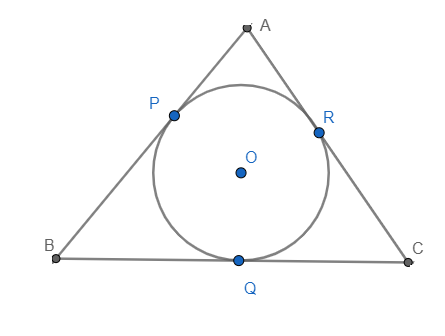QUESTION

# A circle is inscribed in $\Delta ABC$ touching AB, BC, and AC at P, Q, and R, respectively. If AB=10cm, AR=7cm and CR=5cm. Find the length of BC.

Hint: Start by drawing a neat diagram followed by using the theorem that the tangents to a circle from a given point outside the circle are equal.

First, we will draw a neat diagram of the situation given in the question.We know that the tangents drawn from a given point to the circle are equal. And it is clear from the figure that AP and AR are tangents drawn from point A, BP, and BQ are tangents from point B, and CQ and CR are tangents from point C.
$\therefore AP=AR.............(i)$
$\therefore BP=BQ...........(ii)$
$\therefore CR=CQ...........(iii)$
Now it is given in the question that CR=5cm. Therefore, putting the value of CR in equation (iii), we get
$\therefore CR=CQ=5cm$
Now using the figure we can say:
AB=AP+BP
Now we will substitute AP from equation (i). On doing so, we get
AB=AR+BP
It is given in the question that AR = 7cm and AB=10cm. So, putting the values of AR and AB in our equation, we get
10=7+BP
$\Rightarrow BP=3$
Therefore, substituting the value of BP in equation (ii), we get
$BP=BQ=3$
Now from the figure, we can say that BC is the sum of BQ and CQ.
$\therefore BC=BQ+CQ=3+5=8cm$
Hence, we can conclude that the length of BC is 8cm.

Note: The centre of the largest circle that fits inside a triangle is called the incentre and is defined as the meeting point of all three angle bisectors of the triangle. In the above figure, O is the incentre of the $\Delta ABC$.